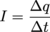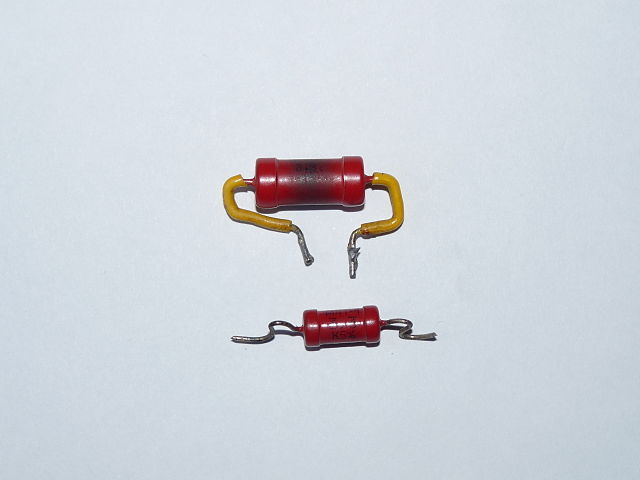Study Guide

## Basic Circuit Elements

All this talk about charged particles and equipotential lines is fine, but when it comes to making light bulbs glow and loudspeakers thump and toasters toast, we need more than just fields and charges. Luckily, generations of electrical engineers have dedicated their lives to building better light bulbs (and, you know, pretty much every other magical modern device), and many of their creations can be described with what we've already learned in this chapter.

The key development in harnessing the power of electricity (aside from Mjolnir) was the electric circuit. "Circuit" is a broad word, but generally includes any sort of defined and constrained path for charged particles to flow around. A common school science project example is a lemon or potato battery, which includes a voltage source to give energy to electrons (the lemon/potato), some wires, and a light. Fruit lux.

A circuit must be made of conductors, materials that allow electrons to flow fairly freely, such as metal. Insulators, like the rubber around copper wire, prevent electrons from flowing. Insulators are nice if you'd like to make a circuit that doesn't contain you as a part of it.

A loop of copper wire connected to a light bulb won't do much, though. All useful circuits need some sort of voltage source to provide power to the rest of the circuit. A voltage source—such as a battery or all those sockets in your wall—adds electric potential to the charge at that point in the circuit.

It's like a giant charge chain lift, and the rest of the circuit is the roller coaster, with charge flying around banked turns and loops and corkscrews. By the time the charge gets back to the voltage source, it needs to be lifted up again if it's going to keep going around the circuit. Charge with no energy to make it through any more of the circuit is at a potential level we call ground (or sometimes common). This marks the zero potential point of a circuit the same way the actual ground marks the zero potential point of gravity.

### Ohm's Law

As charge moves around a circuit, we usually stop thinking of it as a line of individual charges conga dancing their way around our circuit. Instead, we treat it as a continuous stream of charge the same way we look at a river and see "current" instead of "quite a lot of water molecules moving rather rapidly."

Good metaphor, right? Scientists thought so, too, and so the name for a stream of charge is exactly that: current. The current (I) in a wire is equal to the amount of charge (Δ q) that passes through it in time Δt:The SI unit for current is the ampere (A), or amp, where 1 A = 1 C/s. Current is defined to be positive in the direction that positive charge moves—this would make perfect sense, except that it is negatively charged electrons that move around in a circuit. This doesn't change anything about how to deal with circuit questions, but it's useful to remember that if our current is going clockwise through a loop of wire, the electrons in the wire are moving counterclockwise.

The amount of current in a simple circuit is going to be dependent on two things: the size of the voltage source (how much energy is being added to the circuit) and the difficulty electrons have moving through the circuit. The wires in a circuit are so conductive that we usually don't even think about them slowing down current, but that also means they can't do any useful work. One way to make the current in a circuit do work is to force it through a material that's more difficult for charge to navigate. Such a material is said to have a high resistance (R), and the little candy-colored circuit elements made of high-resistance material are called, creatively, resistors.Resistance is tiny.
(Source)

The SI unit of resistance is an ohm (Ω), which is equivalent to a volt per amp.*

Voltage, current, and resistance are all united in Ohm's Law, which is the basic principle underlying almost all electric circuits in existence:

V = IR

There are three different—and all completely correct—ways to interpret this:

• The voltage drop across a resistor is equal to its resistance times the current flowing through it.
• The current in a wire is equal to the voltage applied to it divided by the resistance of the wire or anything connected to it.
• The resistance of an object is equal to the voltage drop across it divided by the current flowing through it.

In the next section, we'll examine how to actually go about making something useful out of this. For now, the important thing to remember is Ohm's Law. In fact, here it is again:

V = IR

### Common Mistakes

Did we mention Ohm's Law? It's V = IR. This applies to only one circuit element at a time—one resistor, for example—but in the next section we see how to mathematically combine multiple elements together in order to use Ohm's Law on more complex circuits.

### Brain Snack

If you ever run out of resistors, feel free to just use cherry Jell-O.

*Interestingly enough, this is exactly equivalent to an inverse second, or 1/s. No credit will be given, however, to answers for time given in 1/Ω. You've been warned.